##Domain of convexity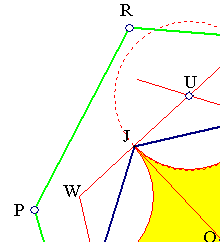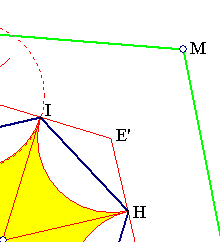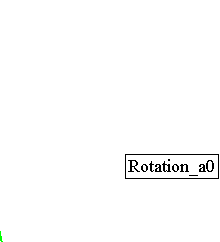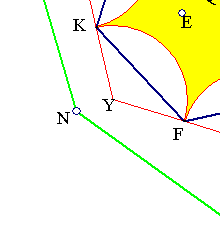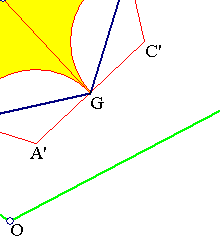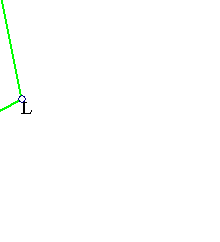Given the regular hexagon p = (HIJKFG) and a point E inside it. Reflect successively E on the sides of the hexagon to get the vertices of the hexagon q = (LMRPNO). For some positions of E q is convex. The symmetric about the center of p, bounded by circular arcs, yellow domain, is the convexity domain of q. For all points outside this domain the polygon q is non-convex. The area of q becomes maximum when E coincides with some vertex of p.
One can ask about generalizations to other polygons. Look at Domain_of_Convexity_quad.html , for a related figure and a hint to solve the problem for convex polygons p.